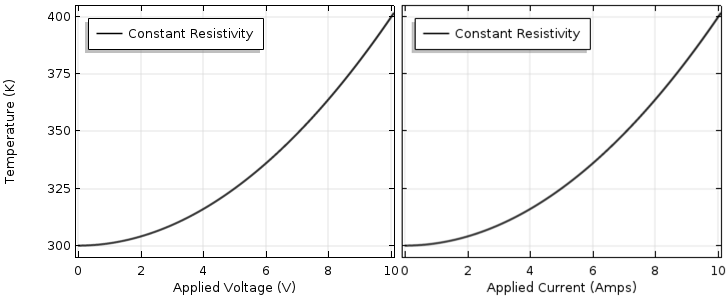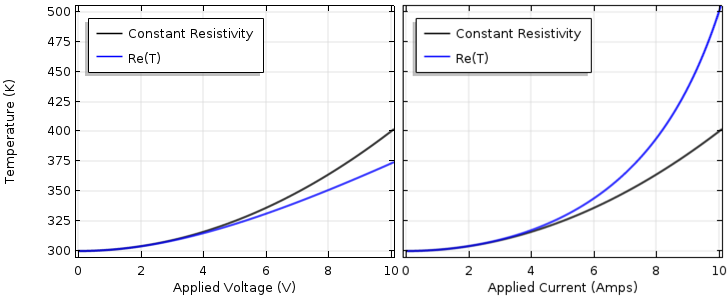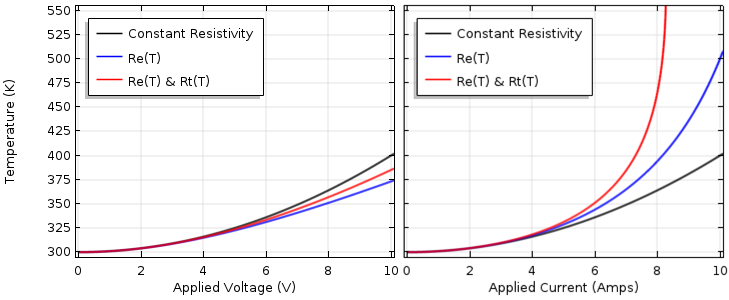# 电热耦合分析中常见的误区

2018年 4月 17日

### 了解欧姆定律和电阻热### 将材料非线性引入简单集总模型

R_e = \rho_0(1+\alpha_e(T-T^e_0))

T = T_{ambient} + (V^2 /\rho_0(1+\alpha_e(T-T^e_0))) R_t

T = T_

{ambient} + I^2 \rho_0(1+\alpha_e(T-T^e_0)) R_tR_t = r_0(1+\alpha_t(T-T^t_0))

T = T_{ambient}
+ V^2 r_0(1+\alpha_t(T-T^t_0))/\rho_0(1+\alpha_e(T-T^e_0))

T = T_

{ambient}
+ I^2 \rho_0(1+\alpha_e(T-T^e_0))/r_0(1+\alpha_t(T-T^t_0))### 后续操作

#### 评论 (3)

##### 留言##### 翔 高
2020-06-05##### kai zhang
2020-06-16 COMSOL 员工2022-11-07